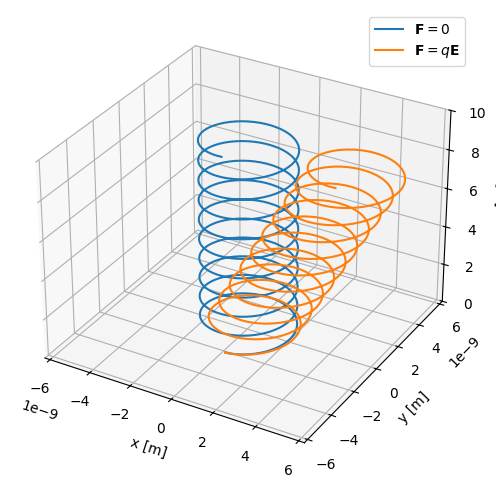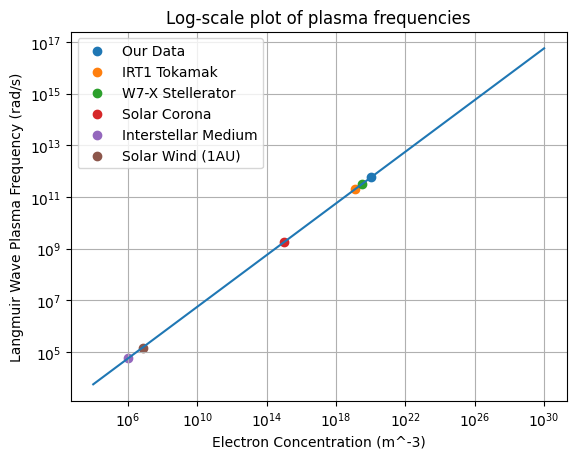# Frequency Plasma Parameters (plasmapy.formulary.frequencies)

Functions to calculate fundamental plasma frequency parameters.

## Functions

 gyrofrequency(B, particle[, signed, Z, to_hz]) Calculate the particle gyrofrequency in units of radians per second. lower_hybrid_frequency(B, n_i, ion[, to_hz]) Return the lower hybrid frequency. plasma_frequency(n, particle[, z_mean, to_hz]) Calculate the particle plasma frequency. upper_hybrid_frequency(B, n_e[, to_hz]) Return the upper hybrid frequency.

## Aliases

PlasmaPy provides short-named (alias) versions of the most common plasma functionality. These aliases are only given to functionality where there is a common lexicon in the community, for example has the alias . All aliases in PlasmaPy are denoted with a trailing underscore _.

 oc_(B, particle[, signed, Z, to_hz]) Alias to gyrofrequency. wc_(B, particle[, signed, Z, to_hz]) Alias to gyrofrequency. wlh_(B, n_i, ion[, to_hz]) Alias to lower_hybrid_frequency. wp_(n, particle[, z_mean, to_hz]) Alias to plasma_frequency. wuh_(B, n_e[, to_hz]) Alias to upper_hybrid_frequency.

## Lite-Functions

Much of PlasmaPy’s functionality incorporates Astropy units for user convenience and to mitigate calculation errors from inappropriate units, but this comes at the sacrifice of speed. While this penalty is not significant for typical use, it can become substantial during intensive numerical calculations. Lite-functions are introduced for the specific case where speed matters, but [USER NOTICE] this comes with the reduction of safeguards so a user needs to know what they are doing! For additional details look to the glossary entry for .

 plasma_frequency_lite(n, mass, z_mean[, to_hz]) The "lite-function" version of plasma_frequency.

## Examples